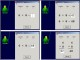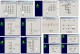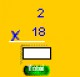Abilities Builder Math Facts 6.6 Learn whole number math facts addition, subtraction, multiplication, division. FreewareAbilities Builder Whole Number Math Facts 7.0 Learn whole number math facts: addition, subtraction, multiplication, division. DemoAbilities Builder Whole Number Math Facts 6.1 Learn whole number math facts: addition, subtraction, multiplication, division. DemoAbilities Builder Math Facts 6.5 Whole number and fraction computation. Number by number problem exercises. DemoiFacts 1.0.1 iFacts is an easy way for children to drill their math facts. SharewareMath Practice 3.0.1 A variety of simple math fact review activities including facts against a timer, building a number sentence and 11 different "math square" games. FreewareAbilities Builder Fraction Facts 6.6 Whole number and fraction computation. Number by number problem exercises. DemoMul tmath for kids 1 Math puzzles. Interactive education. A lot of math information for teachers, Mat FreewareYoungStar Skills Expert: Math 1.0 Out-of-the-box, the software provides 12 math skills activities including Number Basics, Addition Facts, Subtraction Facts, Addition or Subtraction, Counting by Multiples, Multiplication Facts, Division Facts, Quick Quads, Advanced Addition, Advanced Subtraction, Advanced Multiplication, and Advanced Division. DemoNotebook Math One 2.4.1 Addition and subtraction . Shareware